# Law of CosinesHi, and welcome to this video on the Law of Cosines!

Let’s start by taking a look at the Pythagorean Theorem.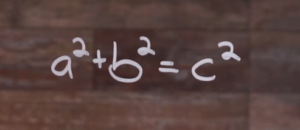It’s that easy-to-remember formula for finding the longest side of a right triangle when we know the length of the other two sides. Wouldn’t it be great if it worked for ALL triangles? That’s what the Law of Cosines does – it allows us to find the third length of any triangle. Here’s the formula:

$$c^2=a^2+b^2-2ab\cdot\text{ cos}(C)$$

As you can see from the end of the formula we need one more piece of information than the Pythagorean Theorem requires – we need the measure of an angle. To be specific, we need the measure of the angle between the two sides we know (sides a & b).

Here’s a triangle we’ll call capital ABC and with the sides labeled lowercase a, b, and c: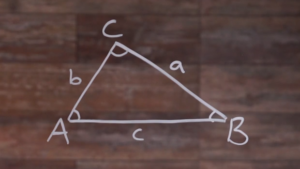As you can see, we’re using lower case letters for sides and upper case letters for angles. So in order to use the Law of Cosines to find side c, we would need to know side b, side a, and $$\angle C$$, which is opposite side c.

Let’s try it out. Look at this problem: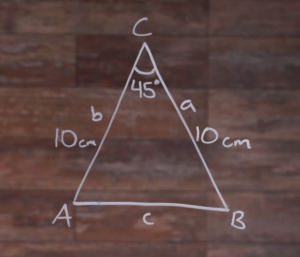First, we need to check to see if we have everything we need to use the Law of Cosines. We have the measure of two sides (a & b, both 10 centimeters) and the measure of the angle between those two sides ($$\angle C$$, which is 45°). That’s all the info we need so let’s plug a, b, and $$\angle C$$ into the formula:

$$c^2=a^2+b^2-2ab\text{ cos}(C)$$
$$c^2=10^2+10^2-2(10)(10)\text{ cos}(45)$$

Be careful with the order of operations here. Evaluate the exponents first, then take the cosine of 45, like this:

$$c^2=100+100-200(0.70710678)$$
$$c^2=200-144.42$$
$$c^2=55.58$$
$$c≅7.46\text{ cm}$$

When we get to the point where we have $$c^2$$ equal to a number, we take the square root of each side to find that c is approximately 7.46 centimeters. A common sense check of that answer tells us that it’s reasonable since the other two sides measure 10 cm and are opposite larger angles, so our answer should be under 10, which it is.

That’s all there is to using the Law of Cosines for finding the missing side of a triangle. In fact, it’s a bit more versatile than the Pythagorean Theorem because we can label any of the sides a, b and c as long as we label the angle opposite that side A, B, and C.

Let’s look at another problem.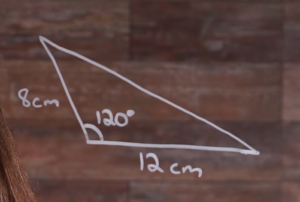We haven’t named any of the sides or angles yet. But we can see that we have been given the measure of two of the sides and the angle between them. So we should label the $$\angle C$$ and the sides a and b, like this: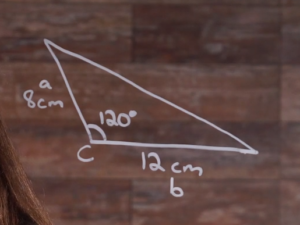Now we can go ahead and plug in the values:

$$c^2=a^2+b^2-2ab\text{ cos}(C)$$
$$c^2=(8)^2+(12)^2-2(8)(12)\text{ cos}(120)$$
$$c^2=64+144-192(-0.5)$$
$$c^2=208+96$$
$$c^2=304$$
$$\sqrt{c^2}=\sqrt{304}$$
$$c≅17.44\text{ cm}$$

Let’s try our common sense check again. Side c is opposite the largest angle so it should be larger than the other sides, and it is. It’s also less than the sum of the other two sides, which it must be in order for this shape to be a triangle.

You might be wondering what would happen if we had a problem with an angle that is 90° and with the two sides around it given.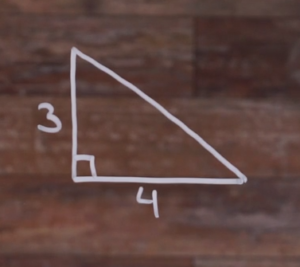Will the Law of Cosines still work? Let’s label the sides and angles: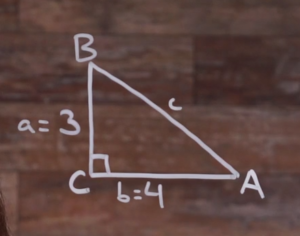Then we’ll plug in our values:

$$c^2=a^2+b^2-2ab\text{ cos}(C)$$
$$c^2=(3)^2+(4)^2-2(3)(4)\text{cos}(90)$$

But watch what happens when we take the cosine of 90:

$$c^2=9+16–2(3)(3)(0)$$

Since the cosine of 90 is 0, that whole final term is 0! So basically it just becomes the Pythagorean Theorem when $$\angle C$$ is 90.

$$c^2=25$$
$$c=5$$

Thanks for watching, and happy studying!

## Practice Questions

Question #1:

In order to solve for the missing third side length of a triangle using the Law of Cosines, the _________________ and the _______________________ need to be provided.

Hypotenuse and the measure of one angle

Measure of all angles and the longest side length

Measure of two sides and the measure of the angle between those two sides

Measure of one side and the measure of one angle

The Law of Cosines is useful for determining the missing third side length of a triangle, or the angles of a triangle when you know all three side lengths. In order to determine the missing third side length of a triangle, you need to know the measure of two sides, and the angle measure between them.

Question #2:

Which equation correctly shows the Law of Cosines?

$$a^2+b^2-2ab\text{cos}(C)=c^2$$
$$c^2+b^2+2ab\text{cos}(C)=a^2$$
$$a^2-b^2-2ab\text{sin}(C)=c^2$$
$$a^3+b^3-2ab\text{cos}(C)=c^3$$

The Law of Cosines is useful for determining the missing third side of a triangle, or the angles of a triangle when you know all three side lengths. The formula resembles the Pythagorean Theorem, except the Law of Cosines works for any triangle, not just right triangles.

Question #3:

Solve for the missing side length $$c$$.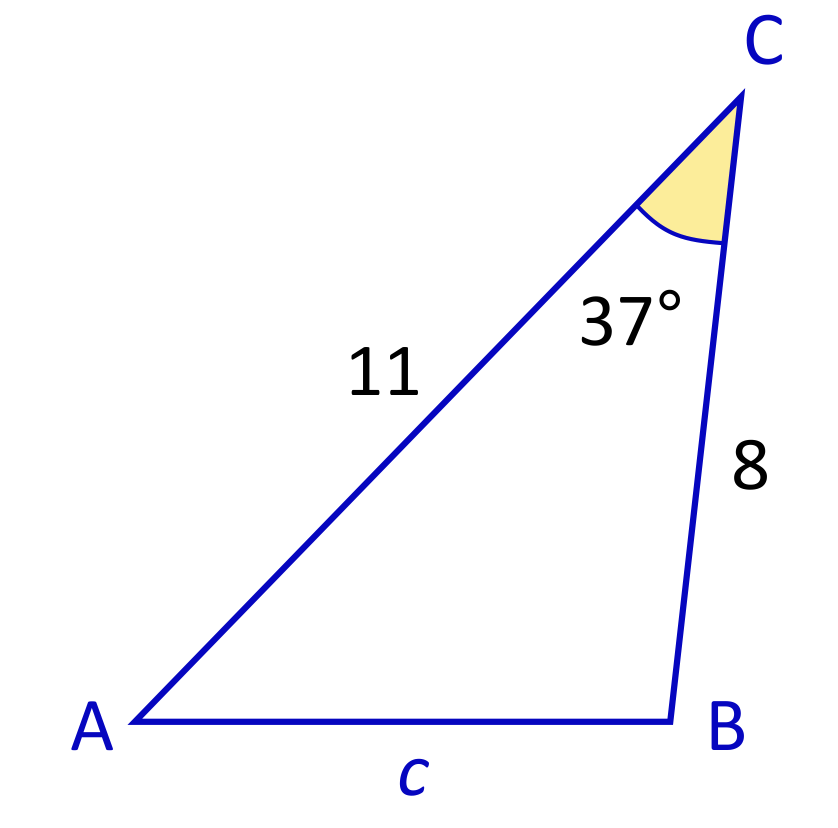8.67

7.67

9.67

6.67

The missing side length c can be calculated using the Law of Cosines. The formula $$c^2=a^2+b^2-2ab\text{cos}(C)$$ becomes $$c^2=8^2+11^2-(2\times8\times11)\text{cos}(37)$$ when the provided values are plugged in. $$\text{cos}(37)$$ is approximately $$0.7986$$, so this can also be plugged into the formula. When the order of operations is carefully followed, $$c^2=8^2+11^2-(2\times8\times11)\text{cos}(37)$$ simplifies to $$c^2\approx44.45$$. Now the square root of both sides can be calculated, which means that $$c\approx6.67$$.

Question #4:

Dan wants to measure the distance across the pond in his backyard. The pond is too shallow to use a boat to measure the distance, so Dan decides to apply his knowledge of the Law of Cosines to make the measurement. He stands back from the pond, and measures 800 feet to one end of the pond, and 900 feet to the other end. The angle between these distances is 70°. What will the distance across the pond be?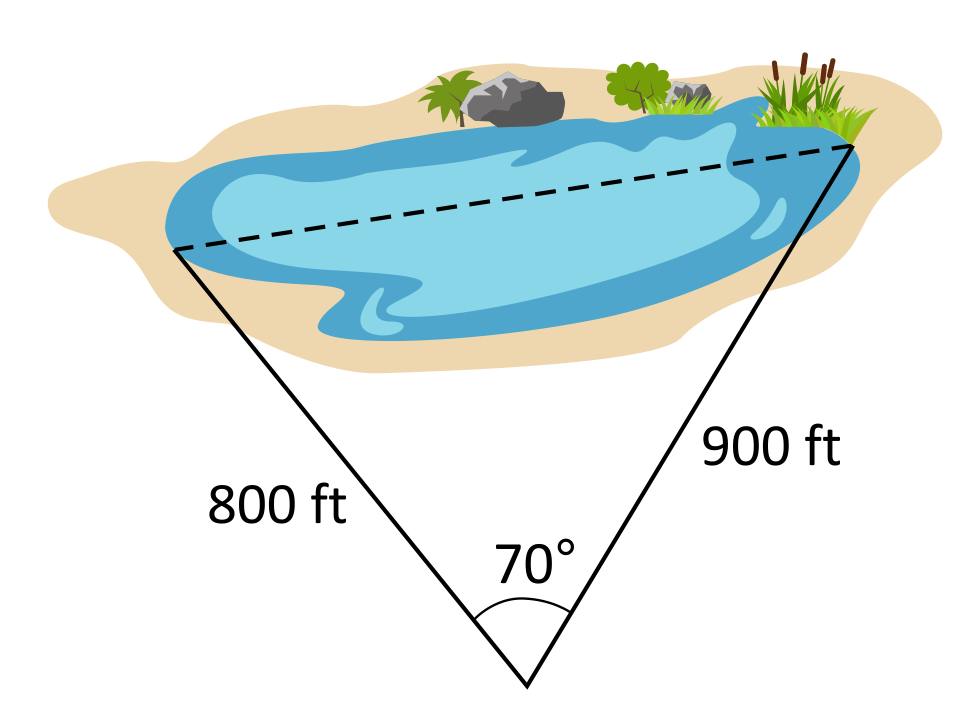900.5 feet

978.5 feet

998.5 feet

1,978.5 feet

The Law of Cosines can be used to determine the distance across the pond because two side lengths are known, as well as the angle between them. The formula $$c^2=a^2+b^2-2ab\text{ cos}(C)$$ becomes $$c^2=800^2+900^2-(2\times800\times900)\text{cos}(70)$$ which simplifies to $$c^2\approx3{,}420.2$$. When the square root of both sides of the equation is calculated, $$c\approx978.5\text{ feet}$$.

Question #5:

Megan is designing a triangular park for her city. She needs one length of the park to be 1 mile, and another length of the park to be 2 miles. The angle between these two sides needs to be 54°. How long will the third side of the triangular park be?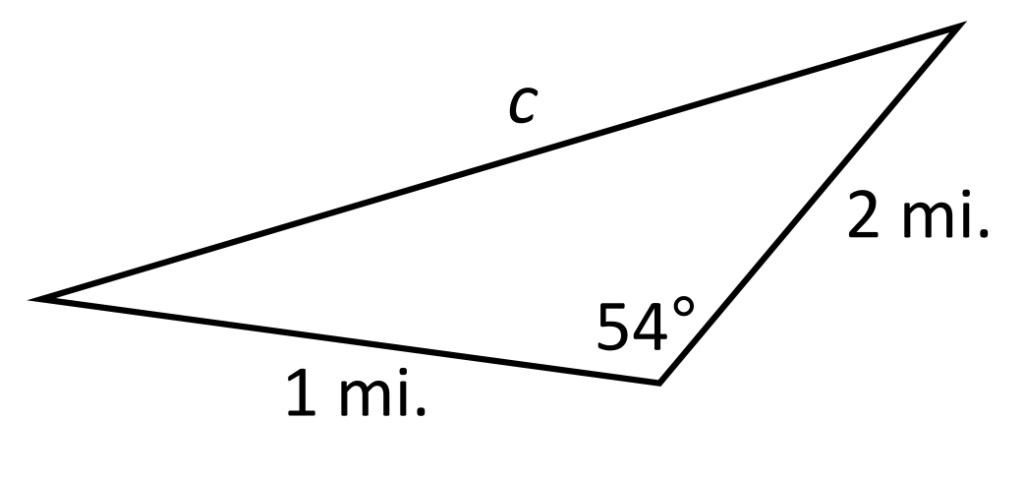1.63 miles

2.63 miles

3.63 miles

4.63 miles

Two side lengths of the park are provided, as well as the angle in between them. This means that the third side of the triangular park can be calculated using the Law of Cosines. The formula $$c^2=a^2+b^2-2ab\text{cos}(C)$$ becomes $$c^2=1^2+2^2-(2\times1\times2)\text{cos}(54)$$ when the provided values are plugged in. When the order of operations are carefully applied, $$c^2=1^2+2^2-(2\times1\times2)\text{cos}(54)$$ simplifies to $$c^2\approx2.65$$. When the square roots of both sides are calculated, $$c\approx1.63\text{ miles}$$.